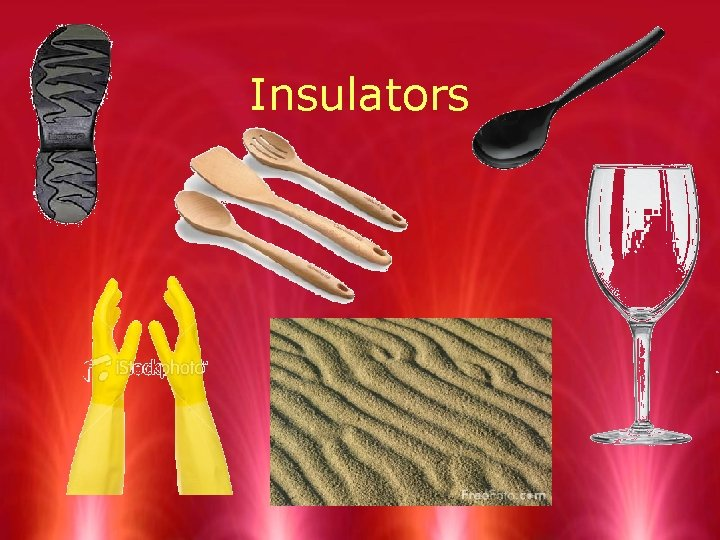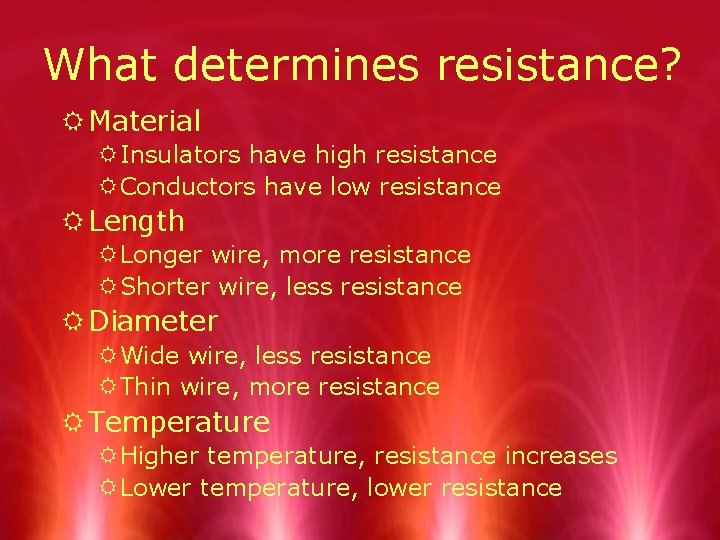# Electricity Electric Current Electric Current R Electric current

• Slides: 12Electricity Electric CurrentElectric Current R Electric current is the continuous flow of electric charges through a material. R Needed to power any electrical device. R Measured in amp (A) R Amps = amount of charge flowing past a given point each second.Circuits RIn order for electric current to exist, a circuit must first exist. RAn electric circuit is a complete, unbroken path through which electric charges can flow.Conductors and Insulators R A conductor is a material through which charges can flow easily. R Electrons are loosely bound R Conduction electrons form an electric current R Carry electric charges R An insulator is a material through which charges cannot flow easily. R Electrons are bound tightly to their atoms R Stop the flow of chargesConductorsInsulatorsRQuia - Insulators & Conductors RThe Blobz Guide - Game 2Voltage R Potential energy is capacity to do work. R Battery provides electrical potential energy R Voltage is the difference in electrical potential energy between two places. R This can also be called potential difference R Voltage causes a current in an electric circuit R Basically, it is the amount of force pushing an electric currentVoltage Source R A voltage source is a device that creates a potential difference in an electric circuit. R The voltage between the terminals is what causes the charges to move R Batteries R GeneratorsResistance RResistance is the measure of how difficult it is for charges to flow through a material. RGreater the resistance, less the current RMeasured in ohmWhat determines resistance? R Material R Insulators have high resistance R Conductors have low resistance R Length R Longer wire, more resistance R Shorter wire, less resistance R Diameter R Wide wire, less resistance R Thin wire, more resistance R Temperature R Higher temperature, resistance increases R Lower temperature, lower resistanceRBrainpop! Current Electricity